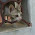## 14 Mar 2012

### Creating a Stratified Random Sample of a Dataframe

Expanding on a question on Stack Overflow I'll show how to make a stratified random sample of a certain size:
```d <- expand.grid(id = 1:35000, stratum = letters[1:10])

p = 0.1

dsample <- data.frame()

system.time(
for(i in levels(d\$stratum)) {
dsub <- subset(d, d\$stratum == i)
B = ceiling(nrow(dsub) * p)
dsub <- dsub[sample(1:nrow(dsub), B), ]
dsample <- rbind(dsample, dsub)
}
)

# size per stratum in resulting df is 10 % of original size:
table(dsample\$stratum)
```

1.1.